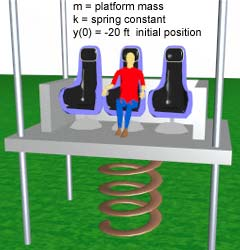Ch 10. Vibrations Multimedia Engineering Dynamics Free Vibs. Undamped Free Vibs. Damped Forced Vibration Energy Method
 Chapter - Particle - 1. General Motion 2. Force & Accel. 3. Energy 4. Momentum - Rigid Body - 5. General Motion 6. Force & Accel. 7. Energy 8. Momentum 9. 3-D Motion 10. Vibrations Appendix Basic Math Units Basic Equations Sections Search eBooks Dynamics Fluids Math Mechanics Statics Thermodynamics Author(s): Kurt Gramoll ©Kurt GramollDYNAMICS - CASE STUDYProblem Parameters Introduction Designers are testing a new idea for an amusement ride. The design is basically a large platform that is on vertical sliders and connected to a spring. The system is subjected to an initial displacement then released. If the designers are not careful, the ride may be to dangerous to use. What is known: mass m = 1,000 slugs spring constant k = 5,000 or 10,000 lb/ft initial displacement y(0) = -20 ft initial velocity y(0) = 0 ft/s Question What is the maximum acceleration the occupants will undergo during the ride? Approach Model the ride as a spring-mass system. Displace the system from its equilibrium position. Draw a free-body diagram. Determine the differential equation of motion using F = ma. Solve the equation of motion for the displacement. Differentiate with respect to time twice to get the acceleration equation.

Practice Homework and Test problems now available in the 'Eng Dynamics' mobile app
Includes over 400 problems with complete detailed solutions.
Available now at the Google Play Store and Apple App Store.